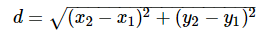# Distance and Midpoint Calculator

User-friendly geometry tool Distance and Midpoint Calculator determines the distance and midpoint for given coordinates effortlessly. You just need to input the coordinate values to get the distance and midpoint in no time.

<form>

Distance and Midpoint Calculator: Finding the distance and midpoint between two points becomes quite easy with our advanced distance and midpoint calculator. Continue reading the article to know about the formulas for distance and midpoint between two points. Apart from that, you will find step by step explanation detailing how to find the distance and midpoint between two points. Use this handy tool to make all your calculations accurate and quick.

## What is distance & Midpoint?

Distance: The distance between two points is usually the length of the line segment connecting them. Remember, the distance between the two points is always positive.

Midpoint: In Maths, the midpoint is defined as the middle point of the line segment. It is equidistant from both the endpoints of the line segment. In fact, it is halfway for the line segment and in other words, it can be referred to as the point that divides a line segment into two equal segments.

### How to find Distance and Midpoint between two points?

The Procedure to evaluate distance and midpoint between two points manually is given below. For the sake of your convenience, we have listed the formulas to evaluate the distance and midpoint for the given coordinates. Use them and make your calculations easy.

Distance between two points

Let the two points (x₁, y₁) and (x₂, y₂) are the coordinates of the endpoints of the hypotenuse. The distance between the two points calculation formula is similar to the right triangle rule, where the squared hypotenuse is equal to the sum of the squares of the other two sides. Formula to find the distance between two points is given byMidpoint

Midpoint is simply the average of each coordinate of the section forming a new coordinate point.

Let the Coordinates be (X1, Y1) and (X2, Y2), and in order to find midpoint simply add the values in the Parentheses and divide each result by 2.

Formula to obtain the midpoint is given as

 (X, Y) = [(X1 + X2)/2, (Y1 + Y2)/2]

where X1, X2 are the coordinates of X-Axis and Y1, Y2 are the coordinates of Y-Axis.

Perform and understand mathematical calculations in seconds taking the help of online tools provided for several concepts  at onlinecalculator.guru

### FAQs on Distance and Midpoint

1.  What is the formula to find Midpoint between two points?

Formula to find Midpoint between two points is (X, Y) = [(X1 + X2)/2, (Y1 + Y2)/2]

2. What is the formula to calculate the distance between two points?

Formula to Calculate distance between two points is given by3. How to find distance and midpoint easily?

You can find distance and midpoint easily taking the help of our Distance and Midpoint Calculator.

4. Where do I get the complete procedure on how to evaluate distance and midpoint between two points?

You can get the complete procedure on how to evaluate distance and midpoint between two points on our page.

Detailed Steps URL

H1 – Finding Distance and Midpoint for Coordinates (4,12) and (3,5)

H2 – Step by Step Solution to find distance and midpoint of Coordinates (4,12) and (3,5)

Internal Link Text – Free Online Distance and Midpoint Calculator to calculate distance and midpoint of the Coordinates (4,12) and (3,5) i.e. 7.07107 and (3.5, 8.5) with an elaborate explanation.

1. How to Calculate the distance and midpoint of (4,12) and (3,5) easily?

You can calculate the distance and midpoint of (4,12) and (3,5) by placing the given inputs in their respective formulas provided above.

2. What is the distance between two points (4,12) and (3,5)?

Distance between the two points (4,12) and (3,5) is 7.07107

3. What is the midpoint of (4,12) and (3,5)?

The midpoint of (4,12) and (3,5) is (3.5, 8.5).

SEO Title:  Distance and Midpoint Calculator to find distance, midpoint of (4,12) & (3,5)

Meta Description: Distance and Midpoint Calculator evaluates the distance and midpoints between two points (4,12) & (3,5) i.e. 7.07107 and (3.5, 8.5) effortlessly in no time.